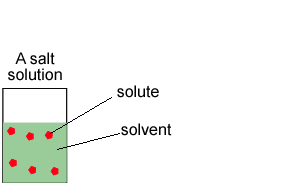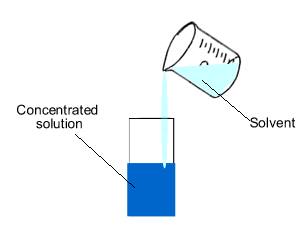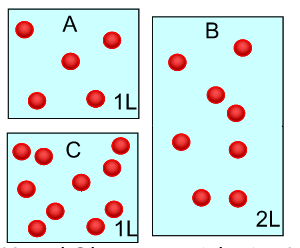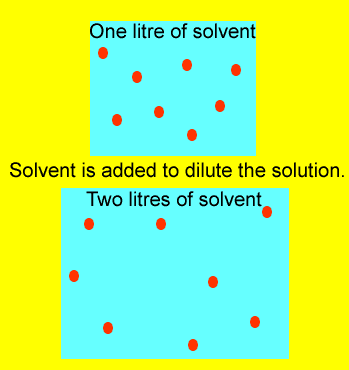Dilution When a solution has solvent, in most cases it is water, added to it, it said to be diluted.By adding water we simply increase the volume in which the solute particles are floating in. We decrease the concentration of the solution.Concentration is the amount of substance, solute, in a given volume of solvent.The number of mole in a given volume of a solution of known concentration is given by the expression Mole = concentration X volume. Look at the image on the right. The number of mole of solute(red balls) remain the same if we dilute (add solvent) the solution. So we can generate the expression below. C1 X V1 = mole = C2 X V2 C1 = initial concentration V1 = initial volume C2 = diluted concentration V2 = larger volumeLets look at an example. What volume of water should be added to 200mls of a 0.1M NaOH solution in order to change the concentration to 0.05M NaOH. What the question is asking is to find the amount of water that must be added to 200mls of a 0.1M solution in order to drop the concentration down to 0.05M. Firstly - Use the formula C1 X V1 = C 2 X V2 Where V1 = 0.2litres , C1 = 0.1 and C2 = 0.05 V2 = (0.2 X 0.1)/ 0.05 = 0.4 litres. Now V2 is the total volume but we wish to find just the amount of solvent added to 200mls. Hence 400-200 = 200mls of solvent must be added to the 200mls of a 0.1M NaOH solution in order to dilute it to 0.05 A 500 ml 12M Nacl solution is to be prepared from a 36M NaCl stock solution. How can this be done? Solution Exercises